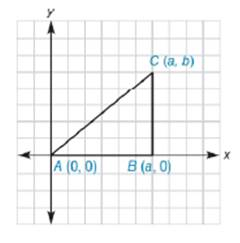Chapter 10.3, Problem 5EElementary Geometry For College St...

7th Edition
Alexander + 2 others
ISBN: 9781337614085

Solutions

Chapter
SectionElementary Geometry For College St...

7th Edition
Alexander + 2 others
ISBN: 9781337614085
Textbook Problem

In Exercises 5 to 10, the real numbers a, b, c, and d are positive.Consider the triangle with vertices at A 0 ,   0 , B a ,   0 , and C a ,   b . Explain why ∆ A B C is a right triangle.To determine

To explain:

ABC is a right triangle.

Explanation

The given figure is shown below.

To prove ABC is a right triangle, we have to prove the following statement.

The midpoint of the hypotenuse of a right triangle is equidistant from the three vertices of the triangle.

The given vertices are A0, 0, Ba, 0, and Ca, b.

The endpoints of the hypotenuse line are A0, 0 and Ca, b.

Find the midpoint of the hypotenuse line.

x1,y1=0, 0

x2,y2=a, b

Formula for midpoint of the line segment joining two points.

M=x1+x22, y1+y22

Substituting the x and y co-ordinates.

M=0+a2, 0+b2

M=a2, b2

Let this midpoint be named as Da2, b2.

Now, find the distance between this midpoint D and A.

x1,y1=Da2, b2

x2,y2=A0, 0

Formula for distance between two points

dDA=x2-x12+y2-y12

Substituting the x and y co-ordinates

dDA=0-a22+0-b22

On solving this,

dDA=-a22+-b22

Squaring,

dDA=a24+b24

dDA=a2+b24

Taking square root,

dDA=a2+b24

dDA=a2+b22

Now, find the distance between this midpoint D and B

Still sussing out bartleby?

Check out a sample textbook solution.

See a sample solution

The Solution to Your Study Problems

Bartleby provides explanations to thousands of textbook problems written by our experts, many with advanced degrees!

Get Started

Find the derivatives of the functions in Problems 1-10. 5.

Mathematical Applications for the Management, Life, and Social Sciences

In Exercises 7-12, refer to the following figure. 12. Which point has a y-coordinate that is equal to zero?

Applied Calculus for the Managerial, Life, and Social Sciences: A Brief Approach

List the first 10 terms of the Fibonacci sequence.

Mathematical Excursions (MindTap Course List)

Expand each expression in Exercises 122. (x22x+1)2

Finite Mathematics and Applied Calculus (MindTap Course List)

Add the following fractions and reduce to lowest terms. 10.

Contemporary Mathematics for Business & Consumers